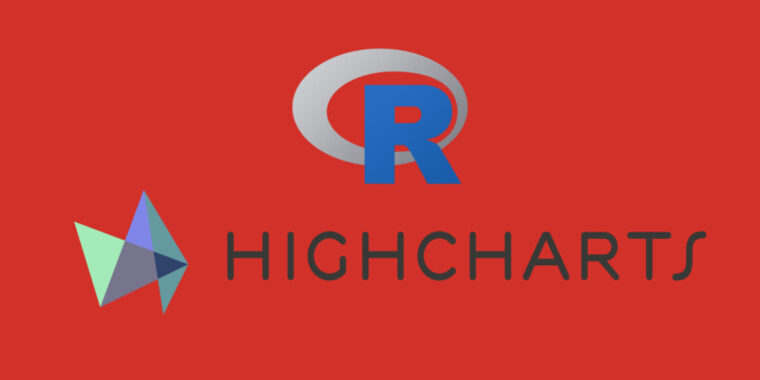### Working with Highcharts JavaScript Syntax in R

Blog Posts Data Science Highcharts R Tutorials0 commentsThis tutorial will show you how to customize and adjust your interactive chart in JavaScript syntax within R.
We will use Highcharter (Highcharts R wrapper) to build the interactive charts and the Highcharts API (in Javascript) to customize the R code.

Well, spoiler alert, it is very easy to use JavaScript in R. All you have to do is to wrap your JavaScript code into the List() method.

Let’s take a look at this demo made in pure Highcharts/JavaScript as an example:

The example includes events and methods such as render() and the dataLabels.formatter function that enable dynamic changes and interaction with the chart.
Now, if you would like to use the same JavaScript code of events and methods in R, all you have to do is to use the list() method, then copy and paste the JavaScript code. In our example, add `events = list()` and `dataLabels = list()` in the R code (source GitHub):

```...
events = list(render = JS("function() {
const chart = this,
data = chart.series.data;
let pointsToProject = [];
(chart.myCustomCircles || []).forEach(function(circle) {
circle.destroy();
});
chart.myCustomCircles = [];
pointsToProject = chart.series.points.filter(function(point) {
return point.flag && point.isInside;
});
// Where to draw a circle
pointsToProject.forEach(function(point) {
const customCircle = chart.renderer.circle(
chart.plotLeft + point.plotX,
chart.plotSizeY + chart.plotTop,
4
)
.attr({
fill: 'red',
zIndex: 2
})
chart.myCustomCircles.push(customCircle);
});
// Where to render the text
if (!chart.myCustomText) {
chart.myCustomText = chart.renderer.text(
',
chart.plotLeft,
35
)
}
chart.myCustomText.attr({
text: 'Number of points with max value: ' + pointsToProject.length,
});
}
"
))
...

series = list(
dataLabels = list(
enabled = TRUE,
formatter = JS("function() {
let visiblePoints = this.series.points.filter(function(point) {
return point.isInside;
}),
max = visiblePoints.reduce(function(acc, cur) {
return Math.max(cur.y, acc);
}, 0);
if (this.y === max) {
this.point.flag = true;
return this.y;
} else {
this.point.flag = false;
}
}
"
)
)```

You can also use a simplified template. For instance, the code below allows you to dynamically change the chart background. This code is a good starting point to create your own advanced configurations:

```library('highcharter')
highchart() % > %
type = "line",
data = list(5, 4, 3, 5)
) % > %
hc_chart(events = list(load = JS("function() {
var chart = this; chart.update({
chart: {
backgroundColor: '#FCFFC5'
}
}); console.log('Updated chart background color!');
}
")
))```

To recap, you can use this customization approach in places where you can write regular JavaScript functions in the available Highcharts API options.

We encourage you to use what you learned here to create interactive charts using R, and you are welcome to leave your questions and comments under this article!